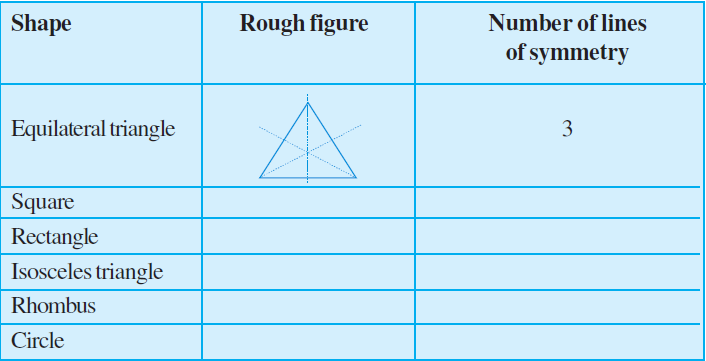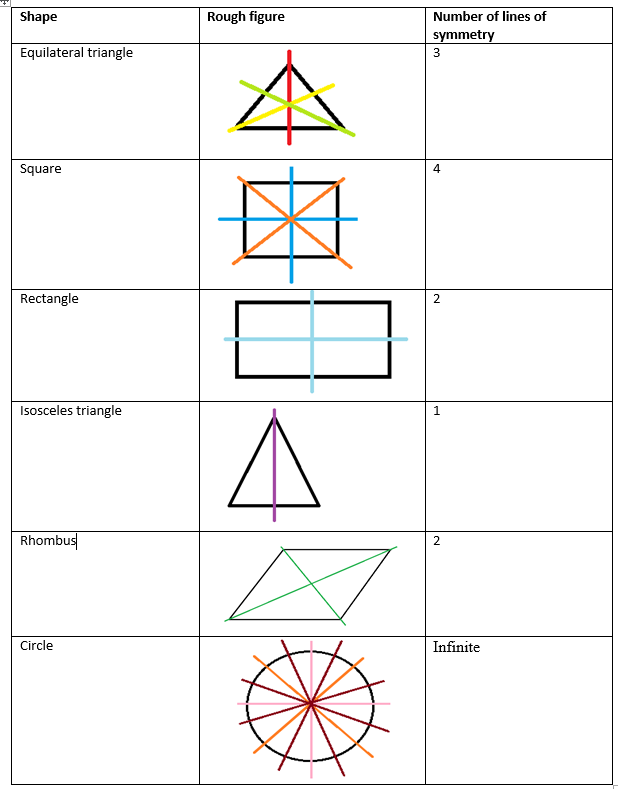# Complete the following table.Solution:

The given table can be completed by the data as followsIn the case of circles, we observe infinite lines. Given here are some lines of symmetry are drawn.

In the same way, we can draw more symmetric lines for the circle.(0)(0)